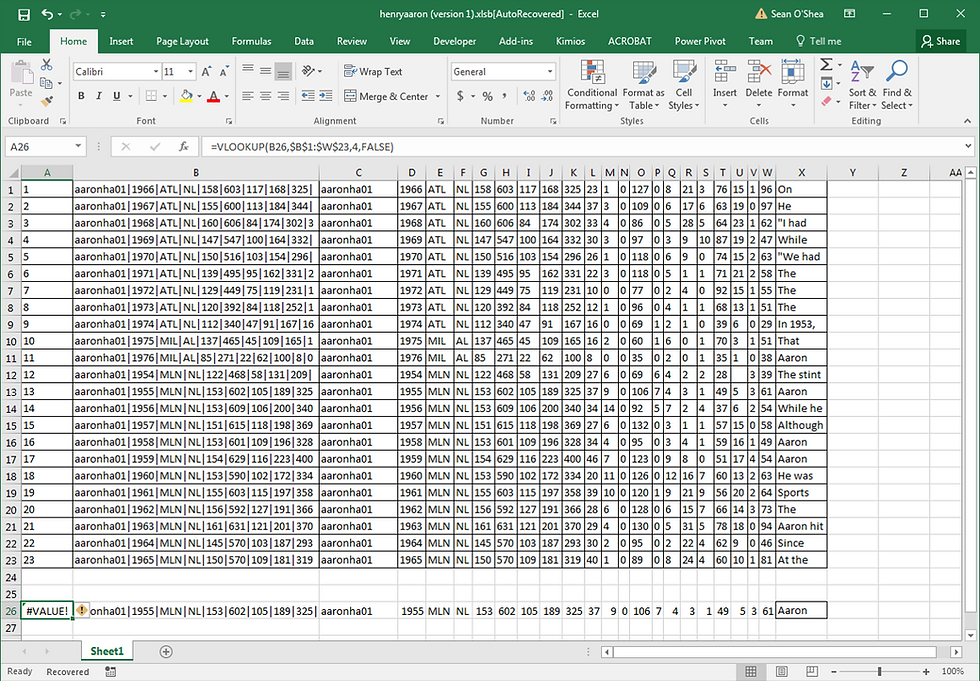top of pageSearch

# Substitute for VLOOKUP when Looking Up Large String

VLOOKUP is a great workhorse for Excel users. However the formula used to find cross referenced entries in an array for a particular value has a serious limitation. If the value you're searching for is greater than 256 characters, the formula will not function and an '# VALUE!' will be returned. See the below example. I am trying to determine if the data in columns C to X on row 26 are matched exactly in the array at C1:X23. In column B, I have combined the values in columns C to X using the macro described in the Tip of the Night for July 28, 2015.

When a VLOOKUP formula is entered in A26, searching for the combined value in B26, the formula fails.The solution to this problem is to use an INDEX MATCH formula like this:

=INDEX(\$A1:\$A\$23,MATCH(TRUE,INDEX(\$B\$1:\$B\$23=B26,0),0))

B26 is the value we're looking for, \$B1:\$B23, is where we search for it, and \$A\$1:\$A\$23 is the range from which a cross referenced value is pulled. So in this example:. . . the control number for the row containing the search for data, '13' listed in column A is pulled by the array formula. As always when using an array formula be sure to press SHIFT + CTRL when entering it so it is surrounded by brackets.

bottom of page